# 4 digit Multiplication Worksheets

• ###### 4 digit Multiplication #15

This set of worksheets are a powerful tool that helps you practice multiplying numbers that have four digits. For example, you might multiply 1234 by 4321. They might seem intimidating at first, but once you get the hang of it, it’s just like any other multiplication problem. And as you practice, you’ll become faster and more accurate, like a true math wizard!

Let’s explore the different types of activities you’ll find in these worksheets:

Straight Multiplication Problems – These are straightforward problems where you have to multiply one 4-digit number by another. You’ll see problems like 1234 x 4321. This helps you practice the basic process of multiplication.

Estimation Problems – In these problems, you’ll be asked to estimate the answer to a multiplication problem. For example, for 1500 x 3000, you might estimate the answer to be around 4,500,000. This type of problem helps you develop number sense and understand the relative size of numbers.

Fill in the Missing Number Problems – In these exercises, you might see a problem like “__ x 2345 = 4690”. Your job is to figure out what number should go in the blank. This helps you think about multiplication in a more flexible way.

True or False Problems – These problems show you a multiplication problem and an answer, and you have to decide if it’s correct. For example, “1234 x 3210 = 3,956,340. True or False?” These problems help you check your understanding and accuracy.

Multiplication with Decimals – In these problems, you might see a question like “12.34 x 43.21”. Multiplying with decimals can be a little tricky, but with practice, you’ll become a pro!

Each type of exercise is like a different type of training in a math gym. They help you understand multiplication, build your speed and accuracy, and teach you to think flexibly about numbers. You’ll learn how to apply multiplication in real-life situations, estimate products, and solve more complex problems involving decimals.

These worksheets are like your training camp for becoming a multiplication master. Each problem you solve, each puzzle you unravel brings you a step closer to that goal. And the skills you gain here won’t just make multiplication easier – they’ll help you in other areas of math, too. It’s like learning how to use a new tool that can help you build all kinds of things.

### How to Perform This Math Skill

To solve a multiplication problem that involves two four-digit numbers, you can use the standard algorithm or long multiplication method. Here’s a step-by-step guide on how to solve a multiplication problem with two four-digit numbers:

Example: Multiply 3,452 by 7,831.

Step 1) Set up the problem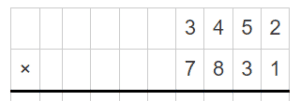Step 2) Multiply the ones place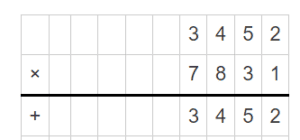Write down the result (4) under the line and carry over the tens place value (2).

Step 3) Multiply the tens place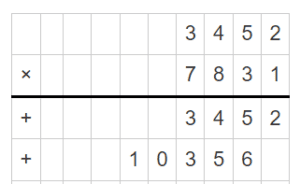Write down the result (8) in the tens place under the line and carry over the hundreds place value (7).

Step 4) Multiply the hundreds place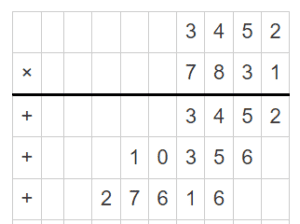Write down the result (0) in the hundreds place under the line and carry over the thousands place value (4).

Step 5) Multiply the thousands place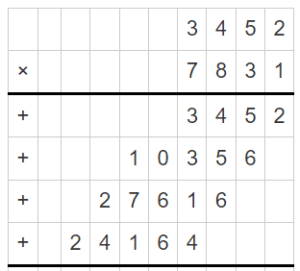+24,520

Write down the result (1) in the thousands place under the line.

Step 6) Add up the partial products

+24,520
———
+27,016,212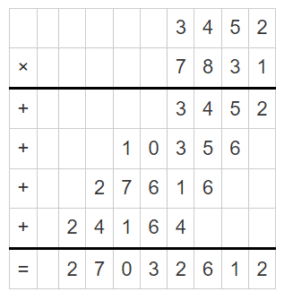The final product is 27,016,212.

Therefore, 3,452 multiplied by 7,831 equals 27,016,212.

By aligning the numbers correctly and performing each multiplication step based on place value, you can solve multiplication problems with two four-digit numbers using the standard algorithm.